# A Quick Introduction to iNEXT.4steps via Examples

#### 2023-09-06

iNEXT.4steps (iNterpolation and EXTrapolation for four steps of biodiversity) is an original R package which provide an easy complete biological analysis computation. In Chao et al. (2020) paper, they proposed a complete biological analysis process:

• STEP1. Sample completeness profiles.

• STEP2. Size-based rarefaction and extrapolation analysis and the asymptotic diversity profile.

• STEP3. Non-asymptotic coverage-based rarefaction and extrapolation analysis.

• STEP4. Evenness among species abundances.

These are the foundation of iNEXT.4steps. Here we will introduce functions about STEP1 and STEP4, particularly. If you want to grasp the functions of STEP2, STEP3 individually, then please search the related paper Hsieh et al. (2016) or the package iNEXT.3D from Anne Chao’s github to get more details. iNEXT.3D contains two major parts:

1. Non-asymptotic diversity:

(1a) Sample-size-based (or size-based) R/E sampling curves: iNEXT3D computes rarefied and extrapolated taxonomic, phylogenetic, or functional diversity estimates under a specified sample size. This type of diversity curve plots the estimated diversity with respect to sample size.

(1b) Sample-coverage-based (or coverage-based) R/E sampling curves: iNEXT3D computes rarefied and extrapolated taxonomic, phylogenetic, or functional diversity estimates under a specified sample coverage. This type of diversity curve plots the estimated diversity with respect to sample coverage.

1. Asymptotic diversity: asymptotic taxonomic, phylogenetic or functional diversity estimate with q-profile, time-profile, and tau-profile.

In iNEXT.4steps package, we provide other four functions for users to calculate and visualize the related biological statistics: Completeness and Evenness for calculation, and ggCompleteness and ggEvenness for visualization. The most comprehensive function iNEXT4steps gathers iNEXT3D, AO3D, Completeness and Evenness into a summary table and a figure. In this document, we will give a quick introduction demonstrating how to run these functions. Detailed information about these function settings is provided in the iNEXT.4steps manual, which will be submitted to [CRAN]. The theoretical basis about these biological statistics can be obtained from the following inference: (http://chao.stat.nthu.edu.tw/wordpress/paper/135.pdf)

## HOW TO CITE iNEXT

If you publish your work based on the results from the iNEXT.4steps package, you should make references to the following methodology paper (Chao et al. 2020):

• Chao, A., Y. Kubota, D. Zelený, C.-H. Chiu, C.-F. Li, B. Kusumoto, M. Yasuhara, S. Thorn, C.-L. Wei, M. J. Costello, and R. K. Colwell (2020). Quantifying sample completeness and comparing diversities among assemblages. Ecological Research, 35, 292-314.

## HOW TO RUN INEXT:

The iNEXT.4steps package will be submitted to [CRAN] and can be downloaded from Anne Chao’s iNEXT.4steps_github using the following commands. For a first-time installation, a related R package (iNEXT.3D) and an additional visualization extension package (ggplot2) must be installed and loaded.

# install_github('AnneChao/iNEXT.3D')
# library(iNEXT.3D)

## install iNEXT.4steps package from CRAN
# install.packages("iNEXT.4steps")  # coming soon

install.packages('devtools')
library(devtools)
install_github('AnneChao/iNEXT.4steps')

## import packages
library(iNEXT.4steps)
library(ggplot2)

An online version of iNEXT.4steps(https://chao.shinyapps.io/iNEXT_4steps/) is also available for users without R background.

## SIMPLE STEPS BRIEF

Step 1: Sample completeness profile

Sample Completeness represent the proportion of observed species in the population (Chao et al., 2020). Usually, the sampling data represents the abundant species in the population so that we will ignore the rare species. Here we will use Turing’s sample coverage theory to reconstruct the population proportion. Besides, sample completeness can correspond to order q, which is an weight index. When order q tends to zero, then we will give more weight to rare species. If order q tends to unity, then we will equally treat each species, which is also called sample coverage at unity. In contrast, if order q tends to larger than unity, we will give more weights to abundant species. By sample completeness, we can easily plot the estimated curve with respect to order q and associated 95% confidence interval.

Step 2.1 and step 3: Size-based and coverage-based Interpolation and Extrapolation

Interpolation and Extrapolation (iNEXT) focuses on three measures of order q: species richness (q = 0), Shannon diversity (q = 1, the exponential of Shannon entropy), and Simpson diversity (q = 2, the inverse of Simpson concentration) (Chao and Jost, 2012; Chao et al. 2014). For each diversity measures, iNEXT uses observed sample to compute expected diversity estimates and associated 95% confidence intervals according two different unit types of rarefaction and extrapolation (R/E):

1. Sample-size-based R/E sampling curves versus diversity in each order q.

2. Coverage-based R/E sampling curves versus diversity in each order q.

For more particular usage about iNEXT, please refer to Hsieh et al. (2016). We won’t introduce details of iNEXT latter.

Step 2.2: Asymptotic diversity profile

Asymptotic Diversity (or called Hill numbers) is a statistic which is used to represent the biological diversity. Its direct meaning is to transform the non-homogeneous distribution into homogeneous distribution (effective number of species). With this quantification criterian, we can easily analysis several communities for different data sources in an objective measure. It usually matches order q to give different weights so that we can focus on rare species or abundant species. When we use observed data to calculate empirical diversity, we usually get an underestimated value. In our package, we will provide an estimated statistic from Chao and Jost (2015), which can imitate a real population accurately. In addition, it can be related to order q index and associated 95% confidence interval.

Step 4: Evenness profile

Evenness is an function to calculate whether a assemblage is uniform or not. We have sorted five main classes according to different transformation by species and diversity (Chao and Ricotta, 2019). In these five classes, they all have range from zero to one. When the value is close to zero, it means that the assemblage tends to uneven. On the contrary, when the value is close one, it means that the assemblage tends to uniform. Evenness considers different order q under each classes. When order q tends to zero, we will focus on rare species. In other sides, when order q tends to far from zero, then we will give more weights on abundant species. If we use observed sampling data to calculate Evenness, we usually have a biased value because of unobserved rare species. But if we try to use asymptotic diversity for calculating Evenness, then we may get a upper bound value. Here, we propose a “standardized coverage” (named Cmax) as a judged criterion. Cmax means that we computes the diversity estimates for the minimum sample coverage among all samples extrapolated to double reference sizes. Under Cmax, we can guarantee the accuracy of Evenness. According to this criterion, we can plot the Evenness curves versus order q and associated 95% confidence interval.

The steps 2.1, 2.2, and 3 can also be promoted to phylogenetic diversity and functional diversity under attribute diversity framework; see Chao et al. (2019, 2021) to get more details.

## DATA FORMAT/INFORMATION

Three types of data are supported:

1. Individual-based abundance data (datatype="abundance"): Input data for each assemblage/site include species abundances in an empirical sample of n individuals (“reference sample”). When there are N assemblages, input data consist of an S by N abundance matrix, or N lists of species abundances.

2. Sampling-unit-based incidence data: There are two kinds of input data.

1. Incidence-raw data (datatype="incidence_raw"): for each assemblage, input data for a reference sample consisting of a species-by-sampling-unit matrix; each element in the raw matrix is 1 for a detection, and 0 otherwise. When there are N assemblages, input data consist of N lists of raw matrices, and each matrix is a species-by-sampling-unit matrix.
2. Incidence-frequency data (datatype="incidence_freq"): input data for each assemblage consist of species sample incidence frequencies (i.e., row sums of the corresponding incidence raw matrix). When there are N assemblages, input data consist of an (S+1) by N matrix, or N lists of species incidence frequencies. The first entry of each column/list must be the total number of sampling units, followed by the species incidence frequencies.

## MAIN FUNCTION: iNEXT4steps()

We first describe the main function iNEXT4steps() with default arguments:

iNEXT4steps(data, diversity = "TD", q = seq(0, 2, 0.2), datatype = "abundance",
nboot = 50, nT = NULL,
PDtree = NULL, PDreftime = NULL, PDtype = "meanPD",
FDdistM = NULL, FDtype = "AUC", FDtau = NULL,
details = FALSE
)
The arguments of this function are briefly described below, and will explain details by illustrative examples in later text.
Argument Description
data
1. For datatype = "abundance", data can be input as a vector of species abundances (for a single assemblage), matrix/data.frame (species by assemblages), or a list of species abundance vectors.
2. For datatype = "incidence_freq", data can be input as a vector of incidence frequencies (for a single assemblage), matrix/data.frame (species by assemblages), or a list of incidence frequencies; the first entry in all types of input must be the number of sampling units in each assemblage.
3. For datatype = "incidence_raw", data can be input as a list of matrix/data.frame (species by sampling units); data can also be input as a matrix/data.frame by merging all sampling units across assemblages based on species identity; in this case, the number of sampling units (nT, see below) must be input.
diversity selection of diversity type: TD = Taxonomic diversity, PD = Phylogenetic diversity, and FD = Functional diversity.
q a numerical vector specifying the diversity orders. Default is (0,0.2,0.4,…,2).
datatype data type of input data: individual-based abundance data (datatype = "abundance"), sampling-unit-based incidence frequencies data (datatype = "incidence_freq"), or species by sampling-units incidence matrix (datatype = "incidence_raw") with all entries being 0 (non-detection) or 1 (detection)
nboot a positive integer specifying the number of bootstrap replications when assessing sampling uncertainty and constructing confidence intervals. Enter 0 to skip the bootstrap procedures. Default is 50.
nT (required only when datatype = "incidence_raw" and input data is matrix/data.frame) a vector of nonnegative integers specifying the number of sampling units in each assemblage. If assemblage names are not specified, then assemblages are automatically named as “assemblage1”, “assemblage2”,…, etc.
PDtree (required only when diversity = "PD"), a phylogenetic tree in Newick format for all observed species in the pooled assemblage.
PDreftime (required only when diversity = "PD"), a vector of numerical values specifying reference times for PD. Default is NULL (i.e., the age of the root of PDtree).
PDtype (required only when diversity = "PD"), select PD type: PDtype = "PD" (effective total branch length) or PDtype = "meanPD" (effective number of equally divergent lineages). Default is meanPD, where meanPD = PD/tree depth.
FDdistM (required only when diversity = "FD"), a species pairwise distance matrix for all species in the pooled assemblage.
FDtype (required only when diversity = "FD"), select FD type: FDtype = "tau_values" for FD under specified threshold values, or FDtype = "AUC" (area under the curve of tau-profile) for an overall FD which integrates all threshold values between zero and one. Default is AUC.
FDtau (required only when diversity = "FD" and FDtype = "tau_values"), a numerical vector between 0 and 1 specifying tau values (threshold levels). If NULL (default), then threshold is set to be the mean distance between any two individuals randomly selected from the pooled assemblage (i.e., quadratic entropy).
details a logical variable to decide whether do you want to print out the detailed value for each plots, default is FALSE.

Here the input data format can be several formats, such as a vector gathered by factors (abundance and incidence_freq), a matrix/data frame with species versus a assemblage (abundance incidence_freq, and incidence_raw), a list of several vectors (abundance and incidence_freq), or a list correspond to a assemblage (incidence_raw). data should comform the format of each datatype. When datatype = "incidence_raw" and class of data is matrix/data frame, user should input nT for each assemblage which represents sampling units.

diversity contains three attributes diversity dimensions: Taxonomic diversity, Phylogenetic diversity, and Functional diversity. User should choose one diversity: TD means Taxonomic diversity, PD means Phylogenetic diversity under a specified reference time (default is root height), and FD means Functional diversity. For the ”Functional diversity”, FDtype = "AUC" consider overall functional diversity which integrates all threshold values between 0 to 1. And FDtype = "tau_values" computes functional diversity under specified thresholds.

When diversity = “PD”, user should input PDtree Newick format data for all observed species. When diversity = “FD”, user should input FDdistM data matrix. Each element of the matrix is the pairwise distance between any two observed species. And the species identification names should be listed as row names and column names of the matrix.

nboot is applied to get confidence interval, which is estimated by bootstrap method. details means a logical setting whether print out the computation value of all figures.

The output of iNEXT4steps will have three parts (if details = TRUE): $summary, $figure, and $details. It may take some time to compute five figures when data size is large or nboot is large. ## Taxonomic Diversity “abundance Data” is used for a random sampling scheme. If the species has aggregation effect, such as trees or plants, then set datatype = “incidence_freq” or datatype = “incidence_raw”. First, we use data Spider to compute taxonomic diversity. ## Abundance-based Datasets Spider were sampled in a mountain forest ecosystem in the Bavarian Forest National Park, Germany (Thorn et al. 2016, 2017). A total of 12 experimental plots were established in “closed forest” stands (6 plots) and “open forest” stands with naturally occurring gaps and edges (6 plots) to assess the effects of microclimate on communities of epigeal (ground-dwelling) spiders. Epigeal spiders were sampled over three years with four pitfall traps in each plot, yielding a total of 3171 individuals belonging to 85 species recorded in the pooled habitat. More details refer to data Source : A mountain forest ecosystem in the Bavarian Forest National Park, Germany (Thorn et al. 2016, 2017). data(Spider) out1 <- iNEXT4steps(data = Spider, diversity = "TD", datatype = "abundance") out1$summary
$STEP1. Sample completeness profiles Assemblage q = 0 q = 1 q = 2 1 Closed 0.61 0.99 1 2 Open 0.77 0.99 1$STEP2. Asymptotic analysis
Assemblage         Diversity Observed Estimator  s.e.   LCL    UCL
1     Closed  Species richness    44.00     72.11 31.24 44.00 133.33
2     Closed Shannon diversity    10.04     10.30  0.41  9.50  11.10
3     Closed Simpson diversity     5.71      5.73  0.24  5.25   6.20
4       Open  Species richness    74.00     96.31 13.03 74.00 121.85
5       Open Shannon diversity    16.34     16.84  0.62 15.62  18.05
6       Open Simpson diversity     9.41      9.46  0.35  8.78  10.14

$STEP3. Non-asymptotic coverage-based rarefaction and extrapolation analysis Cmax = 0.994 q = 0 q = 1 q = 2 1 Closed 55.62 10.18 5.72 2 Open 86.51 16.59 9.43$STEP4. Evenness among species abundances
Pielou J' q = 1 q = 2
Closed      0.58  0.17  0.09
Open        0.63  0.18  0.10
out1$figure[]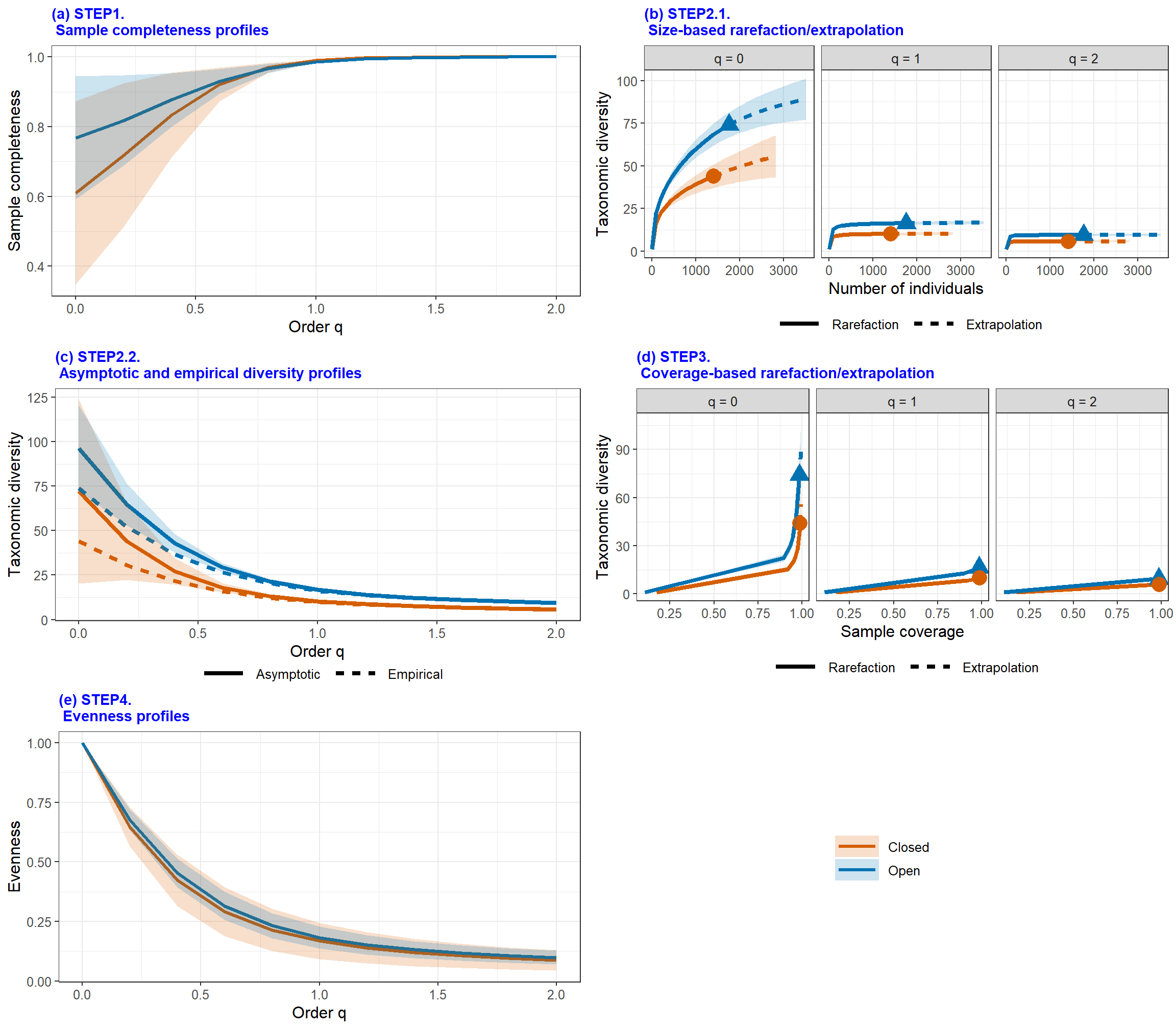$summary lists all biological summaries according to Chao et al. (2020). There are four parts corresponding to each step in the paper. They analysis and explain biological data from different and superimposed side. User can easily compare difference between each assemblages.

$figure visualize the statistics by continuous curves. From the above five figures, iNEXT4stpes provides a standard analysis process from figure (a) to figure (e). User can analyze the process of biodiversity through these figures. $details contains four parts: Sample Completeness, iNEXT, Asymptotic Diversity, Evenness. They are the computing values which are used to plot each figure in $figure. ## Incidence-based Incidence data is matched by incidence-sampling-units. We split a space into several quadrats and only record whether the species is detected or undetected in each quadrat. According to this sampling scheme, incidence_raw data has only value “zero” (undetected) or “one” (detected) in matrix/data frame (species by assemblages). incidence_freq data is the total incidence frequency for each species (i.e., row sums of the corresponding incidence raw matrix). incidence_freq data should contain total sampling units (number of quadrats) in the first row/entry. Remark: The phylogenetic diversity can only select datatype = “incidence_raw” for incidence-based data. Datasets Woody plants are a subset of The National Vegetation Database of Taiwan (AS-TW-001), sampled between 2003 and 2007 within the first national vegetation inventory project (Chiou et al. 2009). Over 3600 vegetation plots, each 20x20-m in area, were set up in various locations in Taiwan, and all woody plant individuals taller than 2 meters were recorded in each plot. For illustration here, we selected only plots belonging to two vegetation types (according to Li et al. 2013): Pyrenaria-Machilus subtropical winter monsoon forest and Chamaecyparis montane mixed cloud forest, sampled in the northern part of Taiwan (in ecoregions 7 and 8 according to Su 1985). data(woody_plants) out2 <- iNEXT4steps(data = woody_plants[,c(1,4)], diversity = "TD", datatype = "incidence_freq") out2$summary
$STEP1. Sample completeness profiles Assemblage q = 0 q = 1 q = 2 1 Monsoon 0.78 0.99 1 2 Upper_cloud 0.78 0.98 1$STEP2. Asymptotic analysis
Assemblage         Diversity Observed Estimator  s.e.    LCL    UCL
1     Monsoon  Species richness   329.00    421.67 20.58 381.34 462.00
2     Monsoon Shannon diversity   145.65    150.15  1.43 147.35 152.95
3     Monsoon Simpson diversity   102.33    103.35  1.11 101.17 105.53
4 Upper_cloud  Species richness   239.00    307.78 18.96 270.62 344.94
5 Upper_cloud Shannon diversity   105.53    110.50  1.72 107.13 113.87
6 Upper_cloud Simpson diversity    71.17     72.23  1.21  69.85  74.60

$STEP3. Non-asymptotic coverage-based rarefaction and extrapolation analysis Cmax = 0.993 q = 0 q = 1 q = 2 1 Monsoon 359.80 147.29 102.67 2 Upper_cloud 278.96 108.52 71.69$STEP4. Evenness among species abundances
Pielou J' q = 1 q = 2
Monsoon          0.85  0.41  0.28
Upper_cloud      0.83  0.39  0.25
out2$figure[]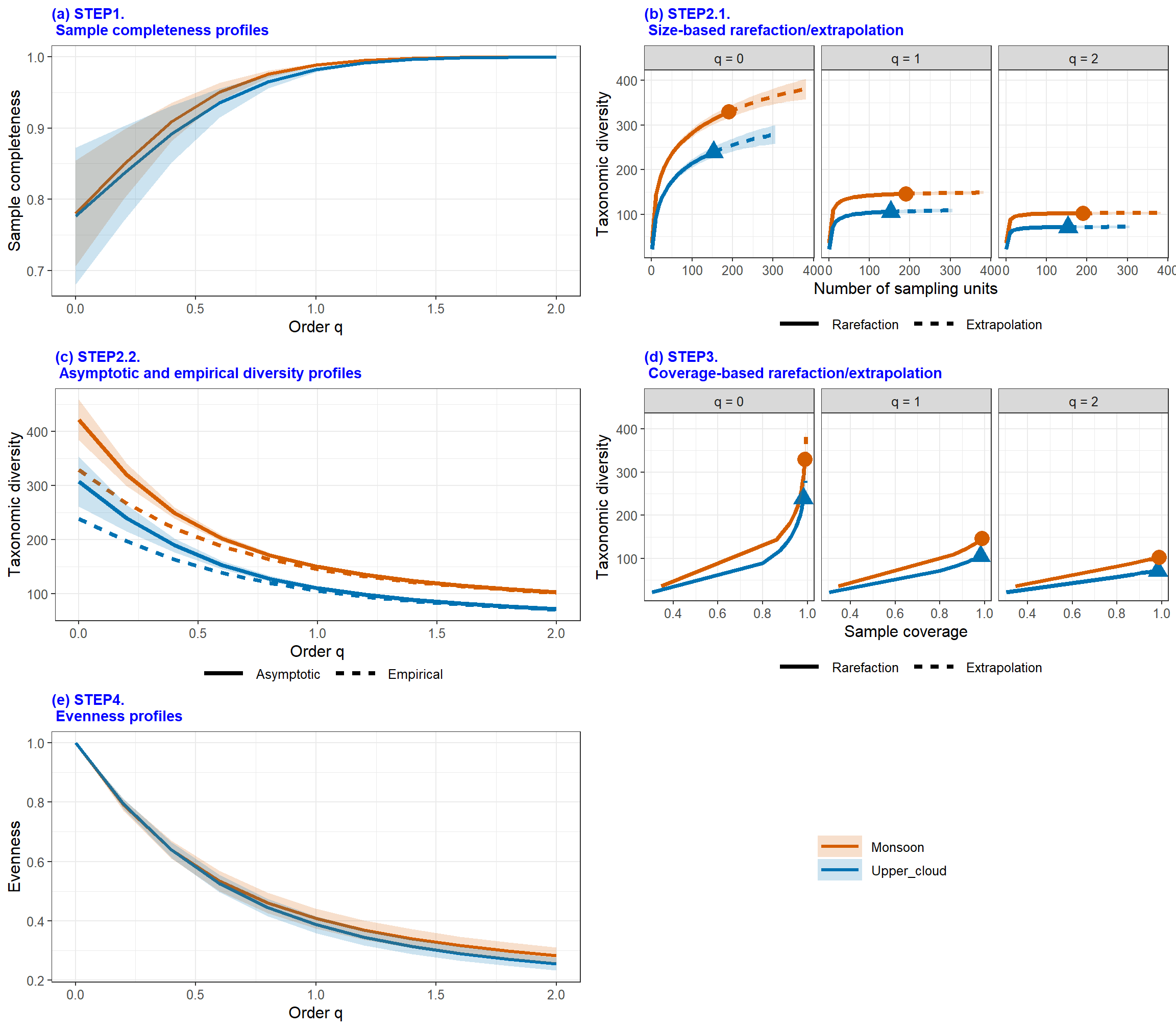## Phylogenetic Diversity Here use abundance data : “brazil” as example. “brazil” data has two main communities: “Edge”, “Interior”. Here we also provide phylogenetic tree data and pairwise distance matrix of “brazil” data to compute phylogenetic diversity and functional diversity. According to following R code, user can get similar output with taxonomic diversity. data(brazil) data(brazil_tree) out3 <- iNEXT4steps(data = brazil, diversity = "PD", datatype = "abundance", nboot = 20, PDtree = brazil_tree) out3$summary
$STEP1. Sample completeness profiles Assemblage q = 0 q = 1 q = 2 1 Edge 0.72 0.94 1 2 Interior 0.69 0.94 1$STEP2. Asymptotic analysis
Assemblage Phylogenetic.Diversity Phylogenetic.Observed Phylogenetic.Estimator s.e.   LCL   UCL Reftime   Type
1       Edge               q = 0 PD                 61.29                  80.03 4.26 71.68 88.38     400 meanPD
2       Edge               q = 1 PD                  5.25                   5.37 0.05  5.27  5.47     400 meanPD
3       Edge               q = 2 PD                  1.80                   1.80 0.01  1.77  1.83     400 meanPD
4   Interior               q = 0 PD                 69.32                  86.38 3.10 80.30 92.45     400 meanPD
5   Interior               q = 1 PD                  5.72                   5.85 0.14  5.59  6.12     400 meanPD
6   Interior               q = 2 PD                  1.91                   1.91 0.04  1.84  1.99     400 meanPD

$STEP3. Non-asymptotic coverage-based rarefaction and extrapolation analysis Cmax = 0.973 q = 0 q = 1 q = 2 1 Edge 71.76 5.32 1.80 2 Interior 80.32 5.80 1.91$STEP4. Evenness among species abundances
Pielou J' q = 1 q = 2
Edge          0.86  0.43  0.21
Interior      0.85  0.40  0.16
out3$figure[]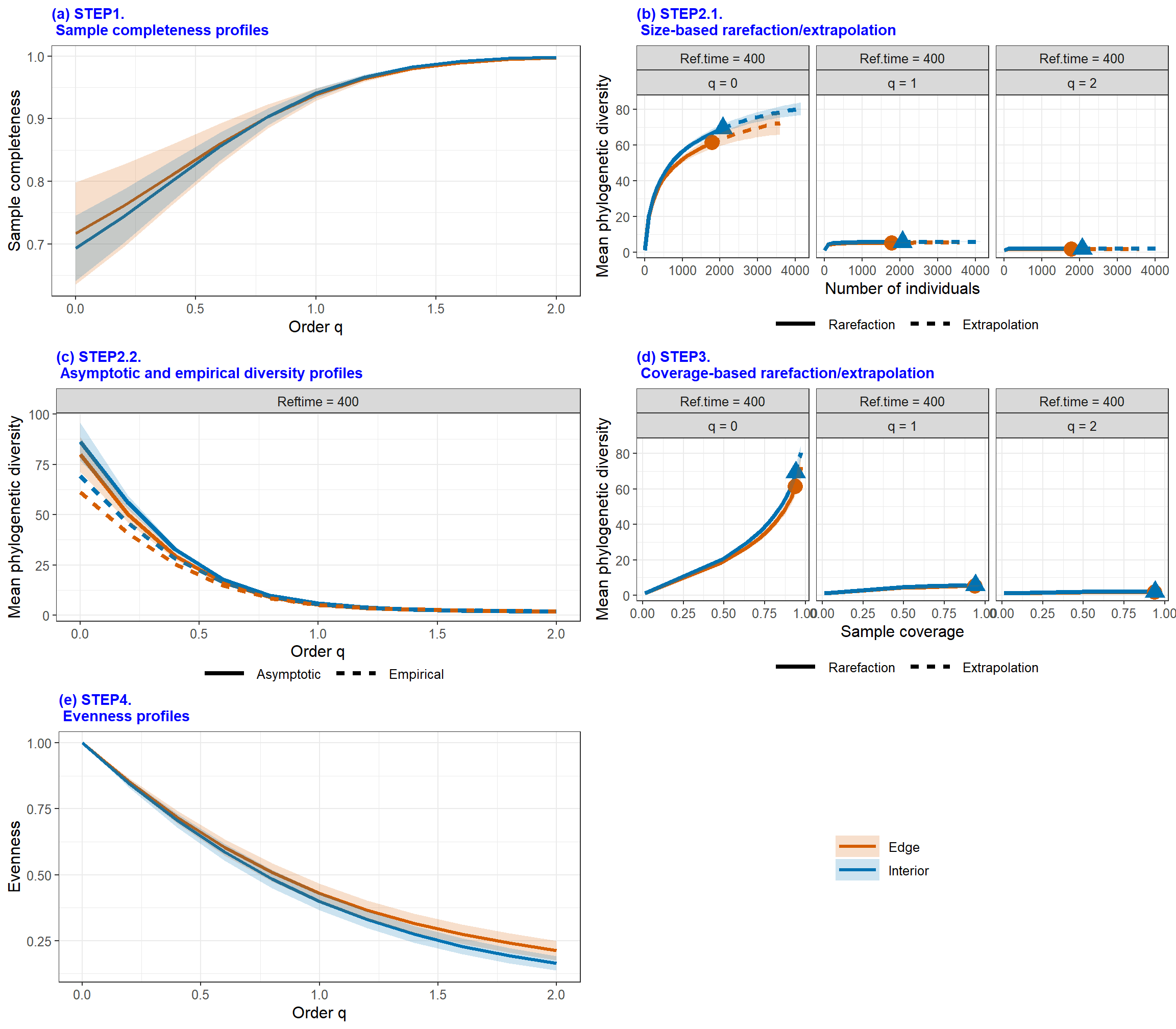## Functional Diversity Here abundance data brazil and its pairwise distance matrix to compute functional diversity. Under FDtype = "tau_values", user can key in FDtau as thresholds. If FDtau is small, all species will tend to different functional groups. If FDtau is large, all species will tend to the same functional group. data(brazil) data(brazil_distM) out4 <- iNEXT4steps(data = brazil, diversity = "FD", datatype = "abundance", nboot = 20, FDdistM = brazil_distM, FDtype = 'tau_values') out4$summary
$STEP1. Sample completeness profiles Assemblage q = 0 q = 1 q = 2 1 Edge 0.72 0.94 1 2 Interior 0.69 0.94 1$STEP2. Asymptotic analysis
Assemblage Functional.Diversity Functional.Observed Functional.Estimator s.e.  LCL  UCL  Tau
1       Edge q = 0 FD(single tau)                6.86                 6.86 0.23 6.86 7.31 0.35
2       Edge q = 1 FD(single tau)                6.52                 6.54 0.14 6.26 6.82 0.35
3       Edge q = 2 FD(single tau)                6.26                 6.28 0.11 6.06 6.50 0.35
4   Interior q = 0 FD(single tau)                5.91                 5.91 0.04 5.91 6.00 0.35
5   Interior q = 1 FD(single tau)                5.19                 5.20 0.06 5.07 5.33 0.35
6   Interior q = 2 FD(single tau)                4.72                 4.72 0.08 4.57 4.88 0.35

$STEP3. Non-asymptotic coverage-based rarefaction and extrapolation analysis Cmax = 0.973 q = 0 q = 1 q = 2 1 Edge 6.86 6.53 6.27 2 Interior 5.91 5.20 4.72$STEP4. Evenness among species abundances
Pielou J' q = 1 q = 2
Edge          0.86  0.43  0.21
Interior      0.85  0.40  0.16
out4\$figure[]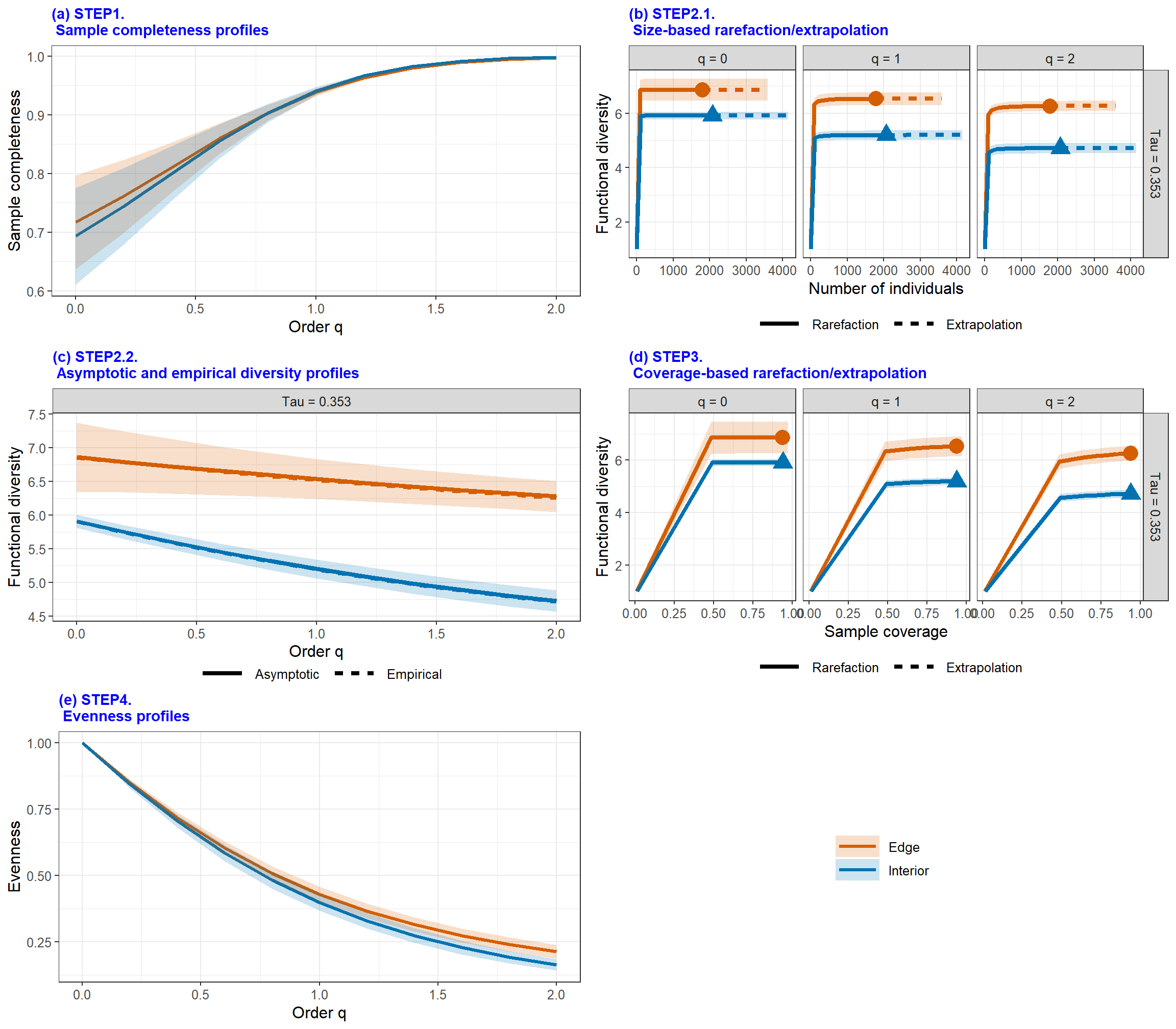## MAIN FUNCTION: Completeness()

iNEXT.4steps provides function Completeness() to compute estimated sample completeness with order q. The arguments is below:

Completeness(data, q = seq(0, 2, 0.2), datatype = "abundance", nboot = 50, conf = 0.95, nT = NULL)
Argument Description
data
1. For datatype = "abundance", data can be input as a vector of species abundances (for a single assemblage), matrix/data.frame (species by assemblages), or a list of species abundance vectors.
2. For datatype = "incidence_freq", data can be input as a vector of incidence frequencies (for a single assemblage), matrix/data.frame (species by assemblages), or a list of incidence frequencies; the first entry in all types of input must be the number of sampling units in each assemblage.
3. For datatype = "incidence_raw", data can be input as a list of matrix/data.frame (species by sampling units); data can also be input as a matrix/data.frame by merging all sampling units across assemblages based on species identity; in this case, the number of sampling units (nT, see below) must be input.
q a numerical vector specifying the diversity orders. Default is (0, 0.2, 0.4,…, 2).
datatype data type of input data: individual-based abundance data (datatype = "abundance"), sampling-unit-based incidence frequencies data (datatype = "incidence_freq"), or species by sampling-units incidence matrix (datatype = "incidence_raw") with all entries being 0 (non-detection) or 1 (detection)
nboot a positive integer specifying the number of bootstrap replications when assessing sampling uncertainty and constructing confidence intervals. Enter 0 to skip the bootstrap procedures. Default is 50.
conf a positive number < 1 specifying the level of confidence interval. Default is 0.95.
nT (required only when datatype = "incidence_raw" and input data is matrix/data.frame) a vector of nonnegative integers specifying the number of sampling units in each assemblage. If assemblage names are not specified, then assemblages are automatically named as “assemblage1”, “assemblage2”,…, etc.

## MAIN FUNCTION: ggCompleteness()

iNEXT.4steps also provides a visualized function ggCompleteness to plot the output from Completeness():

ggCompleteness(output)
Argument Description
output a table generated from function Completeness.

There are two simple examples for functions Completeness and ggCompleteness. One is abundance-based and the other is incidence-based data.

## Abundance-based

Use abundance data Spider to compute sample completeness and plot it.

data(Spider)
out1 <- Completeness(data = Spider, datatype = "abundance")
ggCompleteness(out1)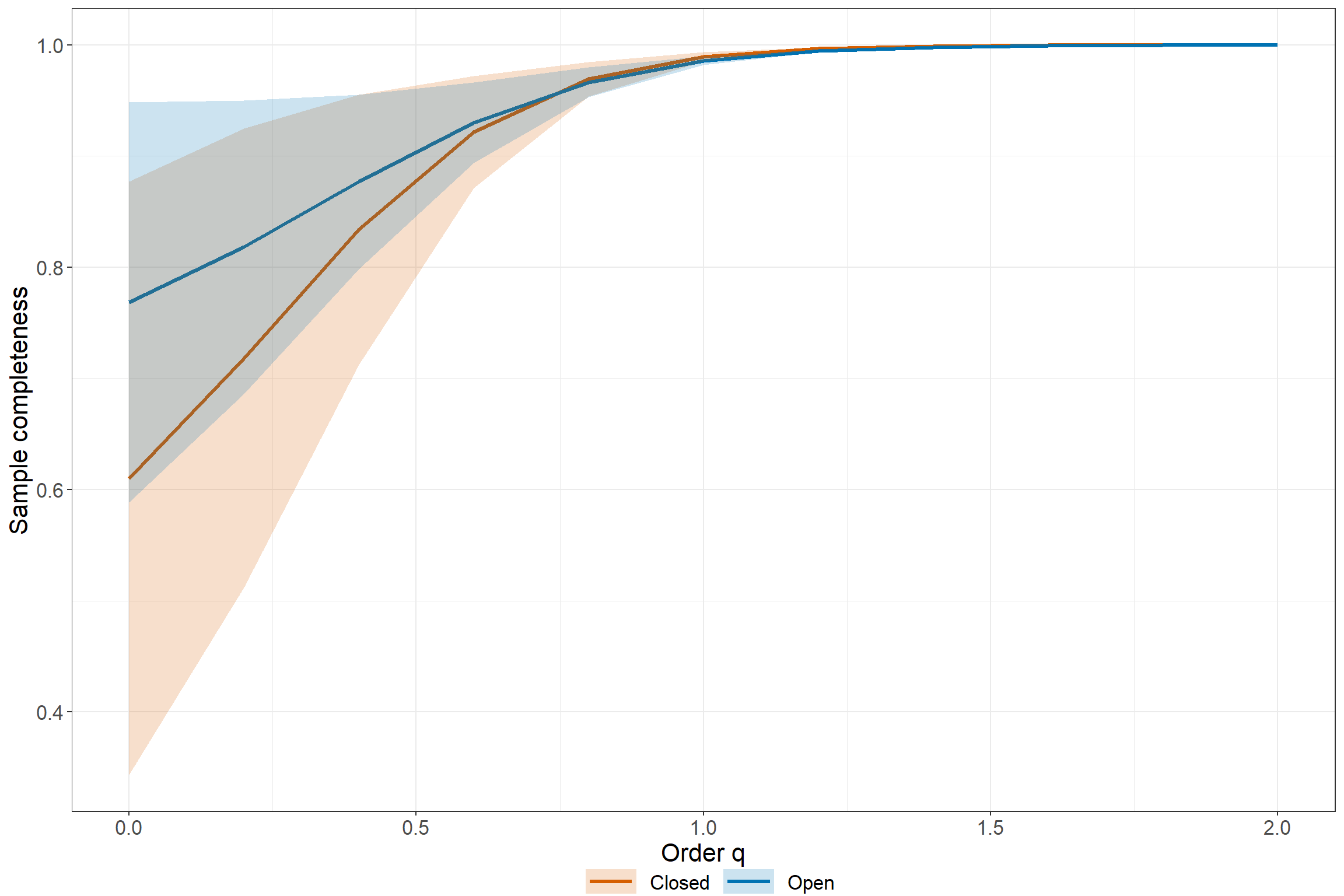## Incidence-based

Use incidence frequency data woody plants to compute sample completeness and plot it.

data(woody_plants)
out2 <- Completeness(data = woody_plants[,c(1,4)], datatype = "incidence_freq")
ggCompleteness(out2)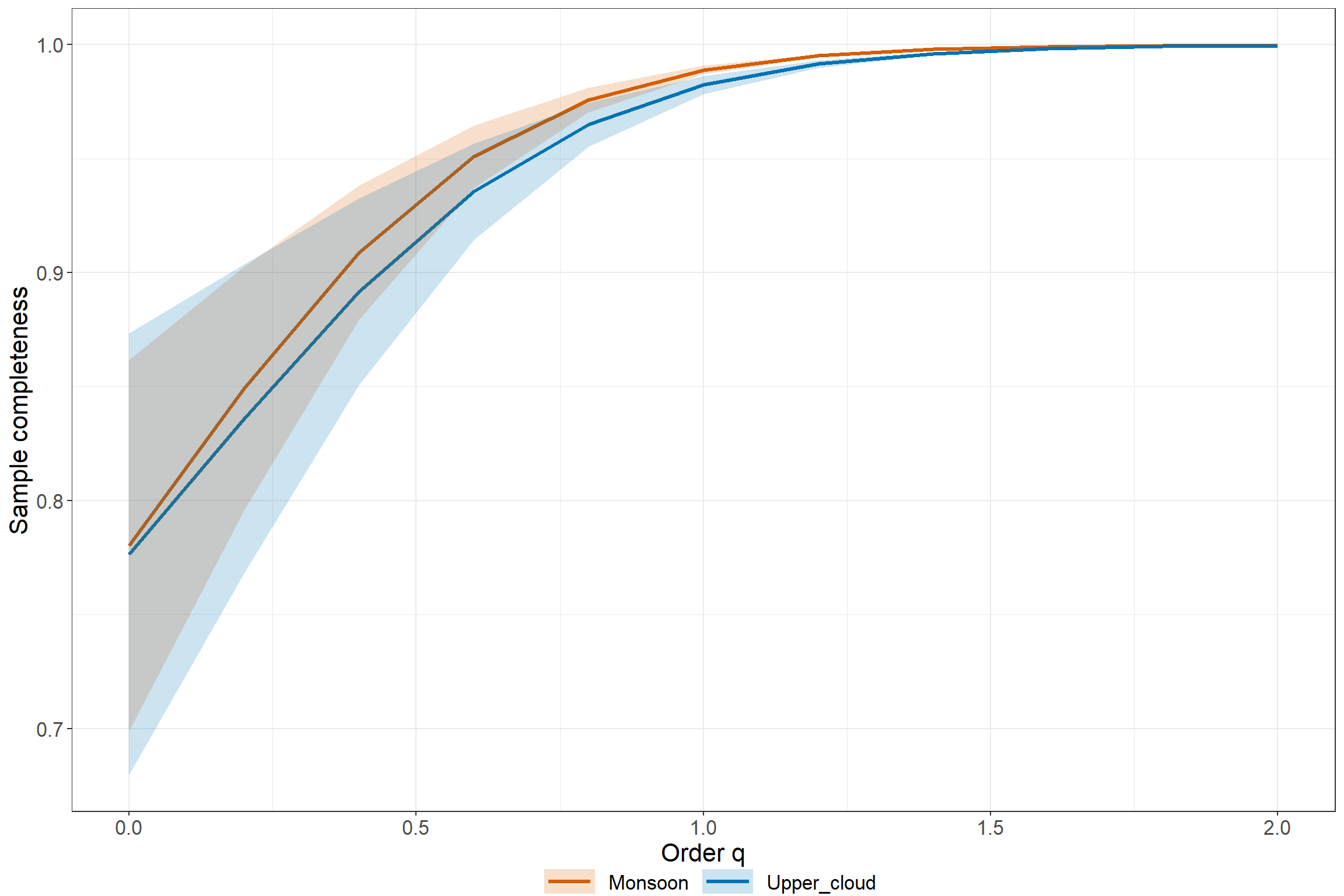## MAIN FUNCTION: Evenness()

iNEXT.4steps provides the function Evenness() to compute observed (empirical) eveness or estimated evenness under specified sample coverage. The arguments is below:

Evenness(data, q = seq(0, 2, 0.2), datatype = "abundance", method = "Estimated",
nboot = 50, conf = 0.95, nT = NULL, E.class = 1:5, C = NULL)
Argument Description
data
1. For datatype = "abundance", data can be input as a vector of species abundances (for a single assemblage), matrix/data.frame (species by assemblages), or a list of species abundance vectors.
2. For datatype = "incidence_freq", data can be input as a vector of incidence frequencies (for a single assemblage), matrix/data.frame (species by assemblages), or a list of incidence frequencies; the first entry in all types of input must be the number of sampling units in each assemblage.
3. For datatype = "incidence_raw", data can be input as a list of matrix/data.frame (species by sampling units); data can also be input as a matrix/data.frame by merging all sampling units across assemblages based on species identity; in this case, the number of sampling units (nT, see below) must be input.
q a numerical vector specifying the diversity orders. Default is (0,0.2,0.4,…,2).
datatype data type of input data: individual-based abundance data (datatype = "abundance"), sampling-unit-based incidence frequencies data (datatype = "incidence_freq"), or species by sampling-units incidence matrix (datatype = "incidence_raw") with all entries being 0 (non-detection) or 1 (detection)
method a binary calculation method with ‘Estimated’ or ‘Empirical’.
nboot a positive integer specifying the number of bootstrap replications when assessing sampling uncertainty and constructing confidence intervals. Enter 0 to skip the bootstrap procedures. Default is 50.
conf a positive number < 1 specifying the level of confidence interval. Default is 0.95.
nT (required only when datatype = "incidence_raw" and input data is matrix/data.frame) a vector of nonnegative integers specifying the number of sampling units in each assemblage. If assemblage names are not specified, then assemblages are automatically named as “assemblage1”, “assemblage2”,…, etc.
E.class an integer vector between 1 to 5. There are five transformation for evenness in Chao and Ricotta (2019). Default is 1:5.
C (required only when method = 'Estimated') a standardized coverage for calculating evenness. If NULL, then this function computes the diversity estimates for the minimum sample coverage among all samples extrapolated to double reference sizes (Cmax).

## MAIN FUNCTION: ggEvenness()

iNEXT.4steps provide a function ggEvenness() to plot the output from Evenness().

ggEvenness(output)
Argument Description
output a table generated from function Evenness.

There are two simple examples for functions Evenness and ggEvenness. One is abundance-based and the other is incidence-based data.

## Abundance-based

Use abundance data Spider to calculate estimated evenness under C = Cmax and plot it.

data(Spider)
out1 <- Evenness(data = Spider, datatype = "abundance")
ggEvenness(out1)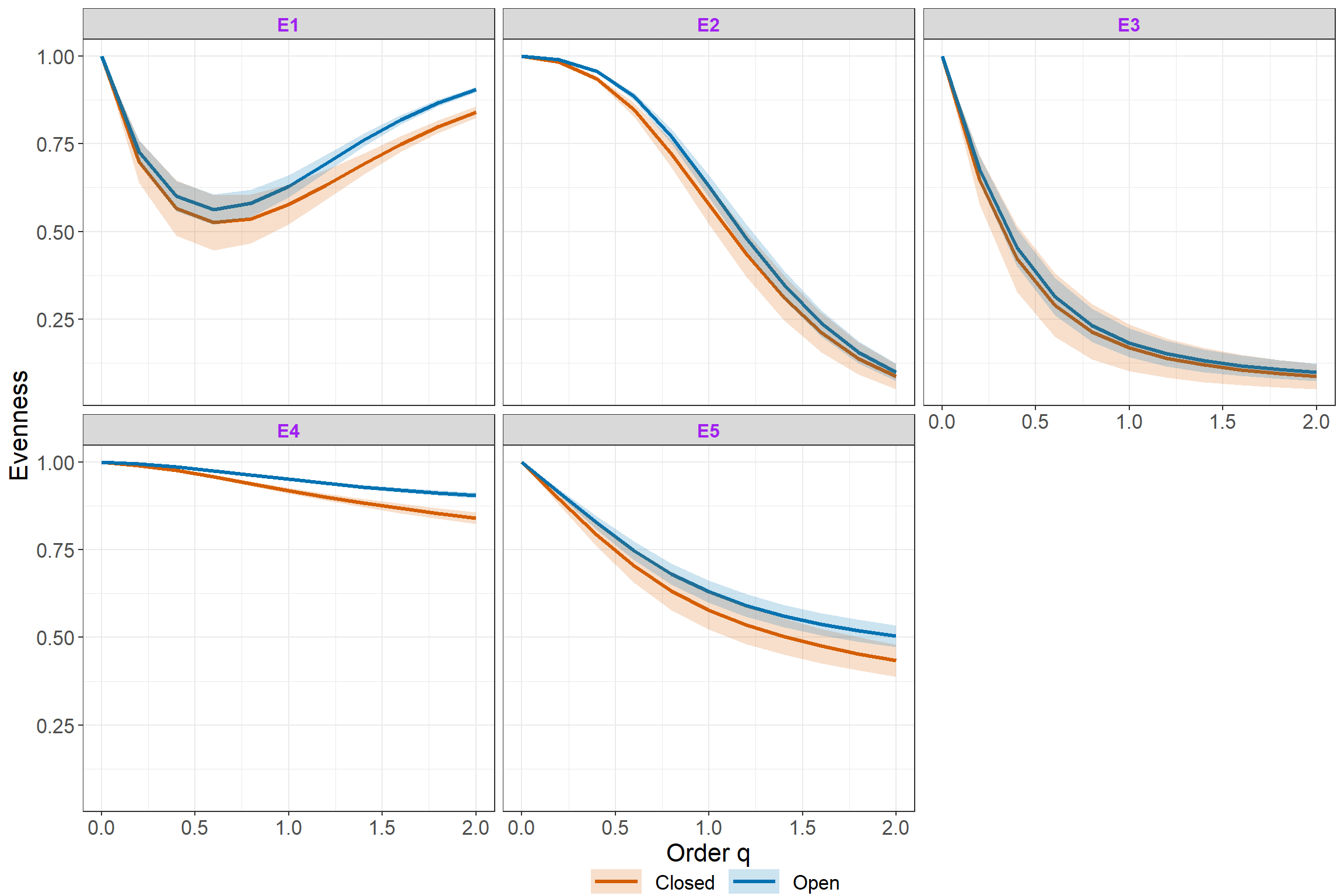## Incidence-based

Use incidence frequency data Woody plants to calculate estimated evenness under C = Cmax and plot it.

data(woody_plants)
out2 <- Evenness(data = woody_plants[,c(1,4)], datatype = "incidence_freq")
ggEvenness(out2)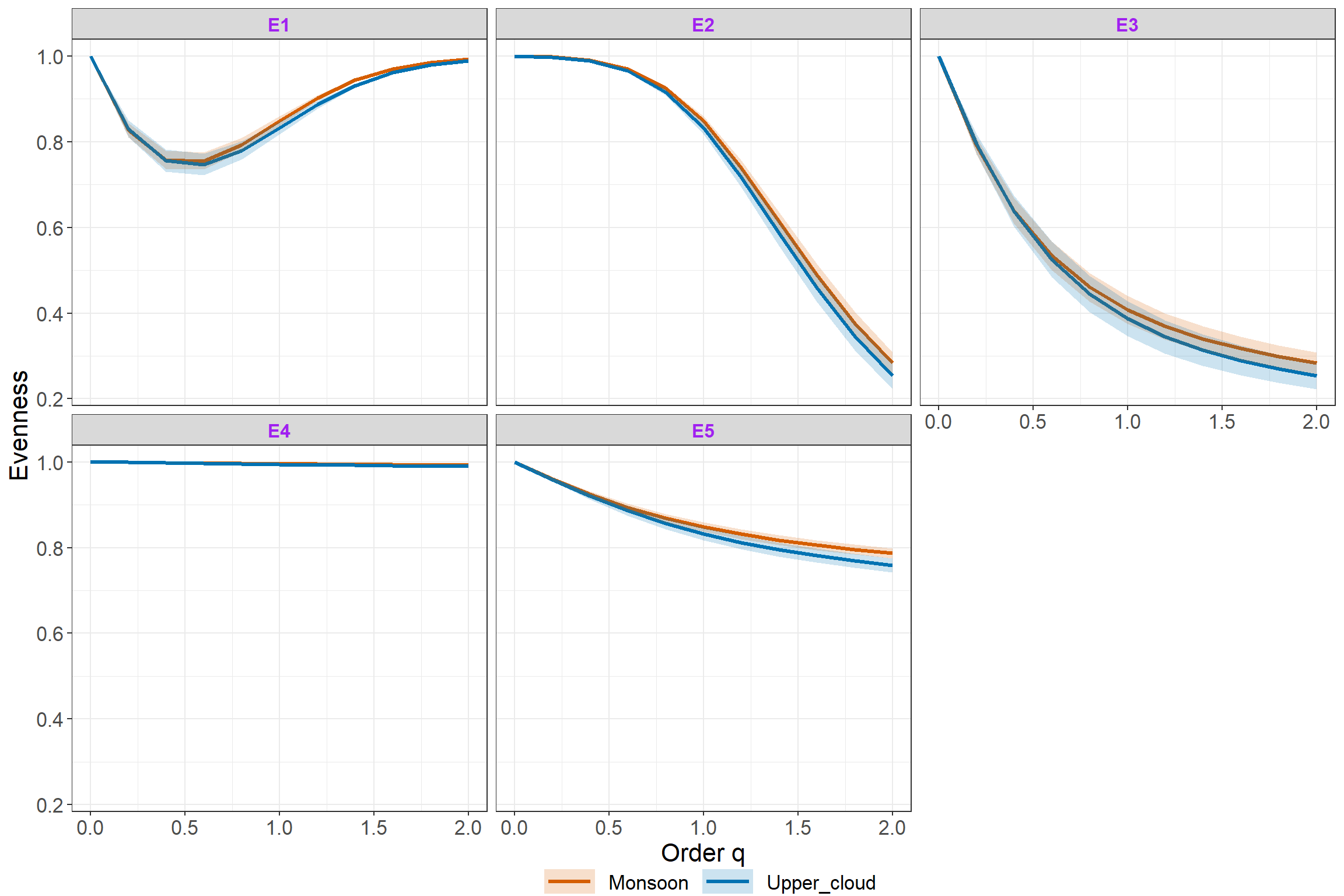The iNEXT.4steps package is licensed under the GPLv3. To help refine iNEXT.4steps, your comments or feedback would be welcome (please send them to Anne Chao or report an issue on the iNEXT.4steps github iNEXT.4steps_github.